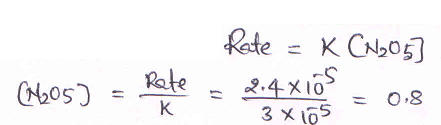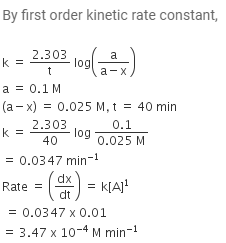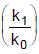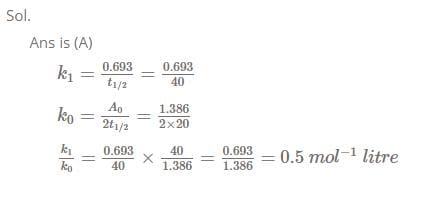JEE  >  MCQ (Previous Year Questions) - Chemical Kinetics (Level 2)

# MCQ (Previous Year Questions) - Chemical Kinetics (Level 2)

Test Description

## 11 Questions MCQ Test Chemistry for JEE Advanced | MCQ (Previous Year Questions) - Chemical Kinetics (Level 2)

MCQ (Previous Year Questions) - Chemical Kinetics (Level 2) for JEE 2022 is part of Chemistry for JEE Advanced preparation. The MCQ (Previous Year Questions) - Chemical Kinetics (Level 2) questions and answers have been prepared according to the JEE exam syllabus.The MCQ (Previous Year Questions) - Chemical Kinetics (Level 2) MCQs are made for JEE 2022 Exam. Find important definitions, questions, notes, meanings, examples, exercises, MCQs and online tests for MCQ (Previous Year Questions) - Chemical Kinetics (Level 2) below.
Solutions of MCQ (Previous Year Questions) - Chemical Kinetics (Level 2) questions in English are available as part of our Chemistry for JEE Advanced for JEE & MCQ (Previous Year Questions) - Chemical Kinetics (Level 2) solutions in Hindi for Chemistry for JEE Advanced course. Download more important topics, notes, lectures and mock test series for JEE Exam by signing up for free. Attempt MCQ (Previous Year Questions) - Chemical Kinetics (Level 2) | 11 questions in 22 minutes | Mock test for JEE preparation | Free important questions MCQ to study Chemistry for JEE Advanced for JEE Exam | Download free PDF with solutions
 1 Crore+ students have signed up on EduRev. Have you?
MCQ (Previous Year Questions) - Chemical Kinetics (Level 2) - Question 1

### Plots showing the variation of the rate constant (k) with temperature (T) are given below. The plot that follows Arrhenius equation is             [JEE 2010]

Detailed Solution for MCQ (Previous Year Questions) - Chemical Kinetics (Level 2) - Question 1

Option (A) is correct. K increases exponentially with a rise in T(K=Ae−Ea​/RT).

The Arrhenius plot is used to study the effect of temperature on reaction rates. The Arrhenius plot can also be used by extrapolating the line back to the y-intercept to obtain the pre-exponential factor, A.

This factor is significant because A=pZ, where p is a steric factor and Z is the collision frequency. The pre-exponential, or frequency, the factor is related to the number of times molecules will hit in the orientation necessary to cause a reaction.

MCQ (Previous Year Questions) - Chemical Kinetics (Level 2) - Question 2

### The rate constant for the reaction 2N2O5 → 4NO2 + O2 is 3.0 × 10–5 sec–1. If the rate is 2.4 × 10–5 mol litre–1 sec–1, then the concentration of N2O5 (in mol litre–1) is           [JEE SCR 2000]

Detailed Solution for MCQ (Previous Year Questions) - Chemical Kinetics (Level 2) - Question 2MCQ (Previous Year Questions) - Chemical Kinetics (Level 2) - Question 3

### If I is the intensity of absorbed light and C is the concentration of AB for the photochemical process AB + hv → AB*, the rate of formation of AB* is directly proportional to           [JEE SCR 2001]

Detailed Solution for MCQ (Previous Year Questions) - Chemical Kinetics (Level 2) - Question 3
In photo initiated primary process rate of reaction is directly proportional to intensity of light uses.
Hence B is the correct answer.
MCQ (Previous Year Questions) - Chemical Kinetics (Level 2) - Question 4

Consider the chemical reaction, N2(g) + 3H2(g) → 2NH3(g). The rate of this reaction can be expressed in term of time derivative of concentration of N2(g), H2(g) or NH3(g). Identify the correct relationship amongst the rate expressions.           [JEE SCR 2002]

MCQ (Previous Year Questions) - Chemical Kinetics (Level 2) - Question 5

In a first order reaction the concentration of reactant decreases from 800 mol/dm3 to 50 mol/dm3 in 2
× 104 sec. The rate constant of reaction in sec–1 is :           [JEE SCR 2003]

MCQ (Previous Year Questions) - Chemical Kinetics (Level 2) - Question 6

The reaction, X → Product follows first order kinetics. In 40 minutes the concentration of X changes from 0.1 M to 0.025 M. Then the rate of reaction when concentration of X is 0.01 M           [JEE SCR 2004]

Detailed Solution for MCQ (Previous Year Questions) - Chemical Kinetics (Level 2) - Question 6MCQ (Previous Year Questions) - Chemical Kinetics (Level 2) - Question 7

Which of the following statement is incorrect about order of reaction ?           [JEE 2005]

MCQ (Previous Year Questions) - Chemical Kinetics (Level 2) - Question 8

Consider a reaction aG + bH → Products. When concentration of both the reactants G and H is doubled, the rate increases by eight times. However, when concentration of G is doubled keeping the concentration of H fixed, the rate is doubled. The overall order of the reaction is           [JEE 2006]

Detailed Solution for MCQ (Previous Year Questions) - Chemical Kinetics (Level 2) - Question 8 AG + bH > product

G H
2x 2x > 8r
2x x >2r

Therefore,
r =[H]^2 [G]
Hence,
order =2+1=3
Correct option,
(D)
MCQ (Previous Year Questions) - Chemical Kinetics (Level 2) - Question 9

Under the same reaction conditions, initial concentration of 1.386 mol dm–3 of a substance becomes
half in 40 seconds and 20 seconds through first order and zero order kinetics, respectively.

Ratioof the rate constants for first order (k1) and zero order (k0) of the reactions is [JEE 2008]

Detailed Solution for MCQ (Previous Year Questions) - Chemical Kinetics (Level 2) - Question 9MCQ (Previous Year Questions) - Chemical Kinetics (Level 2) - Question 10

For a first order reaction A → P, the temperature (T) dependent rate constant (k) was found to follow the equation log k = – (2000) 1/T + 6.0. The pre-exponential factor A and the activation energy Ea, respectively, are           [JEE 2009]

*Multiple options can be correct
MCQ (Previous Year Questions) - Chemical Kinetics (Level 2) - Question 11

For the first order reaction
2N2O5(g) → 4NO2(g) + O2(g)           [JEE 2011]

## Chemistry for JEE Advanced

12 videos|53 docs|118 tests
 Use Code STAYHOME200 and get INR 200 additional OFF Use Coupon Code
Information about MCQ (Previous Year Questions) - Chemical Kinetics (Level 2) Page
In this test you can find the Exam questions for MCQ (Previous Year Questions) - Chemical Kinetics (Level 2) solved & explained in the simplest way possible. Besides giving Questions and answers for MCQ (Previous Year Questions) - Chemical Kinetics (Level 2), EduRev gives you an ample number of Online tests for practice

## Chemistry for JEE Advanced

12 videos|53 docs|118 tests